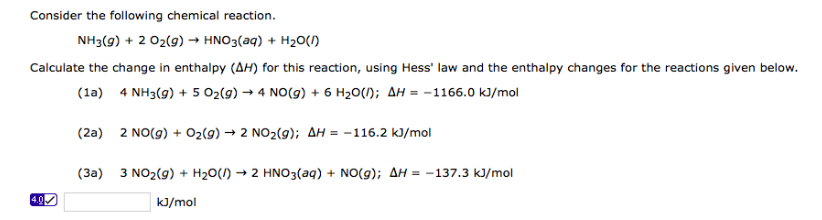# Problem: Consider the following chemical reaction. NH3(g) + 2 O2(g) → HNO3(aq) + H2O(l) Calculate the change in enthalpy (ΔH) for this reaction, using Hess' law and the enthalpy changes for the reactions given below.

🤓 Based on our data, we think this question is relevant for Professor Nair's class at TAMU.

###### FREE Expert Solution###### Problem Details

Consider the following chemical reaction.

NH3(g) + 2 O2(g) → HNO3(aq) + H2O(l)

Calculate the change in enthalpy (ΔH) for this reaction, using Hess' law and the enthalpy changes for the reactions given below.